# solve word problems using addition and subtraction of fractions

##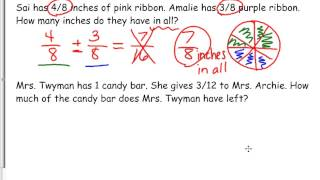By Kathleen Twyman

how to solve a word problem using addition and subtraction of fractions# Addition and subtraction within 10

##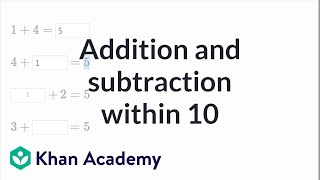By Khan Academy

See how to solve a bunch of addition and subtraction problems with small numbers. All numbers in this video are 10 or less.# Addition using groups of 10 and 100

##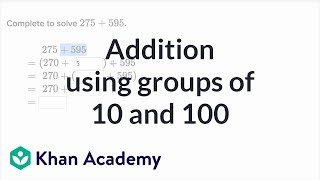By Khan Academy

Learn how to rewrite addition problems to make them easier to solve.# Solving 2-Step Word Problems | Good To Know | WSKG

##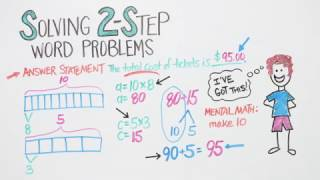By WSKG Public Media

solve two-step word problems using addition, subtraction, multiplication, and division.# Subtracting Fractions in a Word Problem

##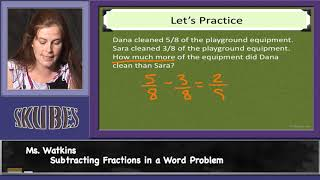By Skubes ed

Solve word problems involving addition and subtraction of fractions referring to the same whole and having like denominators# 2.NBT.9

##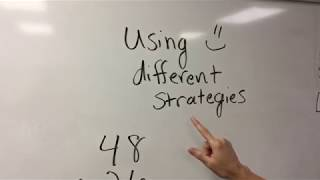By Teresa Plachecki

2nd grade math lesson on using different strategies to solve double digit addition problems.# Multistep Fraction Problems

##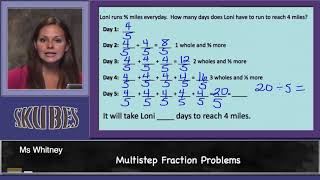By Skubes ed

Solve word problems involving addition and subtraction of fractions referring to the same whole and having like denominators# Algebra for 4th Graders:CCSSM 4.NF.3d

##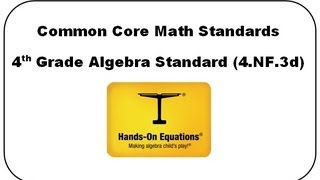By Henry Borenson

Use visual models and equations to represent and solve word problems involving addition and subtraction of fractions (4.NF.3d)# MULTIPLYING FRACTIONS WORD PROBLEMS

##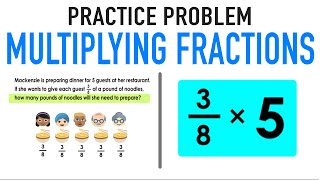By Mashup Math

Solve word problems involving addition and subtraction of fractions referring to the same whole and having like denominators# Telling Time to the Minute

##By Sabrina brown

the nearest minute and measure time intervals in minutes. Solve word problems involving addition and subtraction of time intervals in minutes##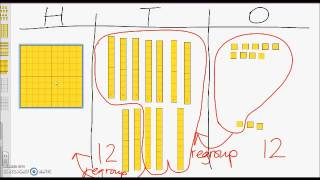By university of Houston mathematics education

The video describes how elementary students can solve an addition word problem regrouping tens and ones.##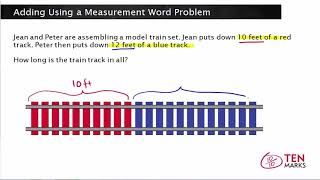By Math mamoth

This lesson presents a measurement word problem that involves addition. A visual is used to write an equation and solve.# 2.NBT.9

##By Teresa Plachecki

2nd grade math lesson on using different strategies to solve double digit addition problems.# Elapsed Time on a timeline

##By Skubes ed

Tell and write time to the nearest minute and measure time intervals in minutes. Solve word problems involving addition and subtraction of time intervals in minutes# [4.NF.3d-1.0] Word Problems: Addition - Common Core Standard

##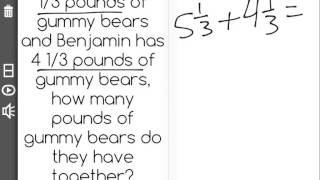By Freckle education

Solve word problems involving addition and subtraction of fractions referring to the same whole and having like denominators, e.g., by using visual fractions models and equations to represent to problem.# Telling Time to the Nearest Minute

##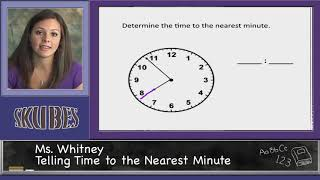By Skubes ed

Tell and write time to the nearest minute and measure time intervals in minutes. Solve word problems involving addition and subtraction of time intervals in minutes, e.g., by representing the problem on a number line diagram.# Adding and Subtracting Angles: 4.MD.7

##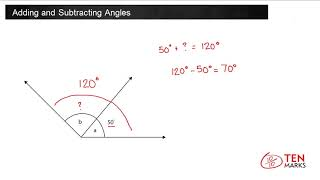By TenmarksAmazon

Recognize angle measure as additive. When an angle is decomposed into non-overlapping parts, the angle measure of the whole is the sum of the angle measures of the parts. Solve addition and subtraction problems to find unknown angles on a diagram in real world and mathematical problems# Addition and subtraction trig identities

##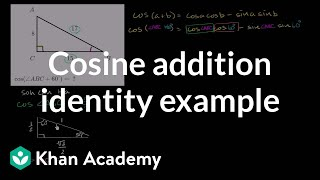By Khan Academy

Sal evaluates the cosine of the sum of 60ï¿½ï¿½ï¿½ï¿½ï¿½ï¿½ï¿½ï¿½ï¿½ï¿½ï¿½ï¿½ and another angle whose right triangle is given. To do this, he must use the cosine angle addition formula.# Addition and subtraction trig identities

##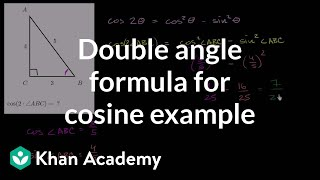By Khan Academy

Sal evaluates the cosine of twice anï¿½ï¿½ï¿½ï¿½ï¿½ï¿½ï¿½ï¿½ï¿½ï¿½ï¿½ï¿½angle whose right triangle is given. To do this, he must use the cosine double-angle formula.##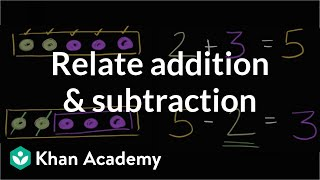By Khan Academy